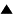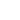# Principles of Reasoning with Uncertainty

Cybernetics and Systems Science have produced some very important principles of information and uncertainty cite{CHR80a,KLG90b}. It should be emphasized that these principles were developed in the context of specific theories which represent information and uncertainty using a particular mathematical formalism of variety, usually probability and thereby stochastic information theory. Instead, we will here describe these principles using the general concept of variety.

These principles operate in the context of a metasystem S' = { S_i }, 1 < i < n. Typically the S_i are various models, descriptions, hypotheses, or other knowledge structures. The simplest measure of the variety of the metasystem S' is given by |S'| = n, the number of subsystems. Also, each S_i has its own variety V_i = V(S_i), e.g. a stochastic entropy.

The principles are:

## Uncertainty Maximization

In inductive reasoning, use all, but no more than, the available information.

The Principle of Uncertainty Maximization has a long and venerable history in inductive reasoning, including Laplace's Principle of Indifference or Principle of Insufficient Reason and Ockham's razor. It provides guidance in choosing a specific subsystem S_i from the metasystem S'.

It can be briefly summarized as follows: in selecting an hypothesis, use no more information than is available. More technically, given some data which constrains the hypotheses S_i such that each S_i is consistent with the data, then choose that hypothesis which has maximal uncertainty V_i.

The most successful application of the Principle is in stochastic systems, where it is the Principle of Maximum Entropy (PME). In combination with Bayesian statistics, the PME has a wide range of important applications as a general method of inductive inference in science, engineering, and philosophy of science cite{SKJ89b}. Like the Law or Requisite Variety, under certain conditions the PME is isomorphic to the 2nd Law of Thermodynamics cite{JAE57}.

## Uncertainty Minimization

In deductive reasoning, lose as little information as possible.

A corresponding Principle of Uncertainty Minimization is a more recent development cite{CHR80a}. It is less a principle of inductive inference or system selection than a principle of systems construction, how to construct a metasystem S' given some systems S_i.

It can be briefly stated as follows: in selecting a metasystem, use all information available. More technically, given some data and a class S = { S^j } of sets of hypotheses S^j = { S^j_i }, all of which are consistent with that data, then let the metasystem S' be that S^j such that V(S^j) is a minimum. Typically V(S^j) = |S^j|, but in a stochastic setting it is possible to consider V(S^j) as a higher order entropy.

Uncertainty Minimization provides a guideline in problem and system formalization. In particular, it relates to our views on meta-foundationalism. Given a situation where we have multiple consistent axiom sets S^j and a requirement to select one, then the Principle should be invoked.

## Uncertainty Invariance

When transforming a system, make the amount of information in the resulting system as close as possible to that in the original.

Like Uncertainty Minimization, this principle is also relatively new, developed by George Klir in 1991 cite{KlG93a}. Its use is to guide the scientist when transforming or translating a system or a problem formulation from one language or representational frame to another.

It can be briefly stated as follows: when doing such a transformation, aim to neither gain nor lose any information. This can, of course, be hard to do if uncertainty and information are represented in different ways in the different representational frames. Therefore, application of this principle is highly problem-specific. For example, uncertainty is measured most generally by the number of available choices, while in probability theory it is measured by entropy, and in possibility theory by nonspecificity. Therefore, when moving a problem formulation from one situation to another, the appropriate measures should be optimized to be as equal as possible.

More technically, given a system S and the metasystem S' = { S_i } of other systems with which we are attempting to model or represent S, the select that S_i such that V(S_i) is as close to V(S) as possible.

 HomeMetasystem Transition TheoryEpistemologyMeta-Cognitive Modelling / Principles of Systems and Cybernetics Up Prev.Next Down